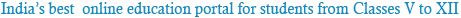### Sample Test Mathematics

1
If A2 – A + I = 0, then the inverse of A is
• 1 A + I
• 2 A
• 3 A – I
• 4 I – A
2
Let R = {(3, 3), (6, 6), (9, 9), (12, 12), (6, 12), (3, 9), (3, 12), (3, 6)} be a relation on the set A = {3, 6, 9, 12} be a relation on the set A = {3, 6, 9, 12}.        The relation is
• 1 reflexive and transitive only
• 2 reflexive only
• 3 an equivalence relation
• 4 reflexive and symmetric only
3
If in a frequently distribution, the mean and median are 21 and 22 respectively, then its mode is approximately
• 1 22.0
• 2 20.5
• 3 25.5
• 4 24.0
4
Let P be the point (1, 0) and Q a point on the locus y2 = 8x. The locus of mid point of PQ is
• 1 y2 – 4x + 2 = 0
• 2 y2 + 4x + 2 = 0
• 3 x2 + 4y + 2 = 0
• 4 x2 – 4y + 2 = 0
5
The system of equations
αx + y + z = α - 1,
x + αy + z = α - 1,
x + y + αz = α - 1
has no solution, if α is
• 1 -2
• 2 either – 2 or 1
• 3 not -2
• 4 1
6
The value of α for which the sum of the squares of the roots of the equation x2 – (a – 2)x – a – 1 = 0 assume the least value is
• 1 1
• 2 0
• 3 3
• 4 2
7
If roots of the equation x2 – bx + c = 0 be two consectutive integers,
then b2 – 4c equals
• 1 -2
• 2 3
• 3 2
• 4 1
8
If the letters of word SACHIN are arranged in all possible ways and these words are written out as in dictionary, then the word SACHIN appears at            serial number
• 1 601
• 2 600
• 3 603
• 4 602
9
Let f be differentiable for all x. If f(1) = - 2 and f′(x) ≥ 2 for x ∈ [1, 6] ,
then
• 1 f(6) ≥ 8
• 2 f(6) < size="2">
• 3 f(6) < size="8">
• 4 None of the above
10
If in a triangle ABC, the altitudes from the vertices A, B, C on opposite sides are in H.P., then sin A, sin B, sin C are in
• 1 G.P.
• 2 A.P.
• 3 Arithmetic − Geometric Progression
• 4 H.P.

Powered by: iVerticals Web Technology Pvt Ltd